﻿ Why helium has NO magnetic field ?

# Helium with "spins" is nonsense

## Quantum mechanical model is unrealistic.

### [ Angular momentum zero + faster-than-light spin = quantum. ]

(Fig.1)  Quantum mechanics gives unreal hydrogen model with spin ↓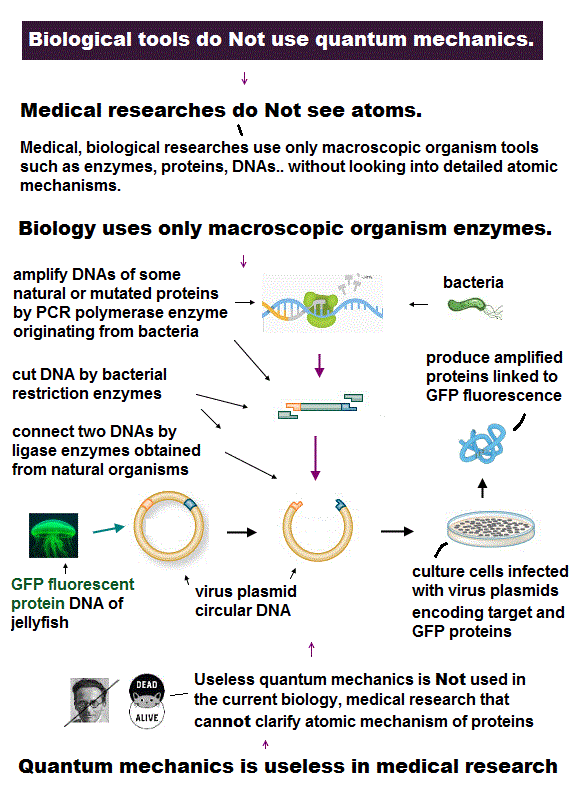Bohr model first succeeded in explaining hydrogen energy levels, and was replaced by quantum mechanics in 1920s.

Surprisingly, quantum mechanics requires that s orbitals (= ground state ) have zero angular momentum ( this p.2 ).  ←Electrons often hit nucleus ?

Magnetic moment of hydrogen atom is known to be equal to Bohr magneton, which was first predicted by Bohr model.

But the quantum mechanical hydrogen with zero angular momentum cannot generate the magnetic moment.

This is the reason why they fabricated artificial spin, which cannot be spinning realistically.

## Electron spinning far exceeds light speed !

### [ Spinning speed of "point"-like electron is much faster than light ! ]

(Fig.2)  Point-like electron ( radius r → 0 ), rotation v → ∞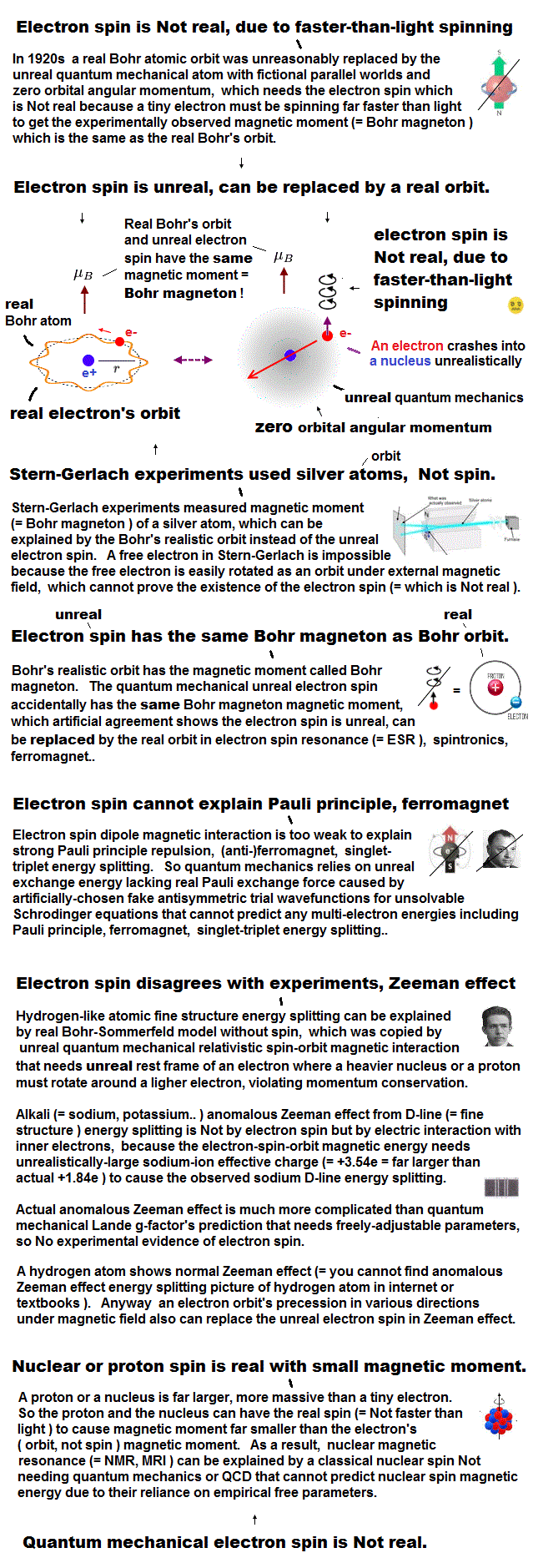Angular momentum is given by mv × r ( v = velocity, r = radius ).
Electron spin also has angular momentum 1/2ħ, they claim

The problem is an electron is very tiny, point-like.
The point-like particle means its radius r is almost zero.

So to get the angular momentum 1/2ħ, the electron spinning must far exceed light speed ( this p.5, this )

So the electron spin lacks reality.
Even Pauli ridiculed the idea of "spinning electron".

But in "s" orbital of Schrodinger's hydrogen, this electron spin is the only generator of magnetic moment.

So they had no choice but to accept this strange spin ( Not as real spinning and speed ).

## Helium by quantum mechanics is unreal

### [ Helium two electrons always crash into nucleus ? ]

(Fig.3)  Quantum mechanics claim electrons have zero angular momentum !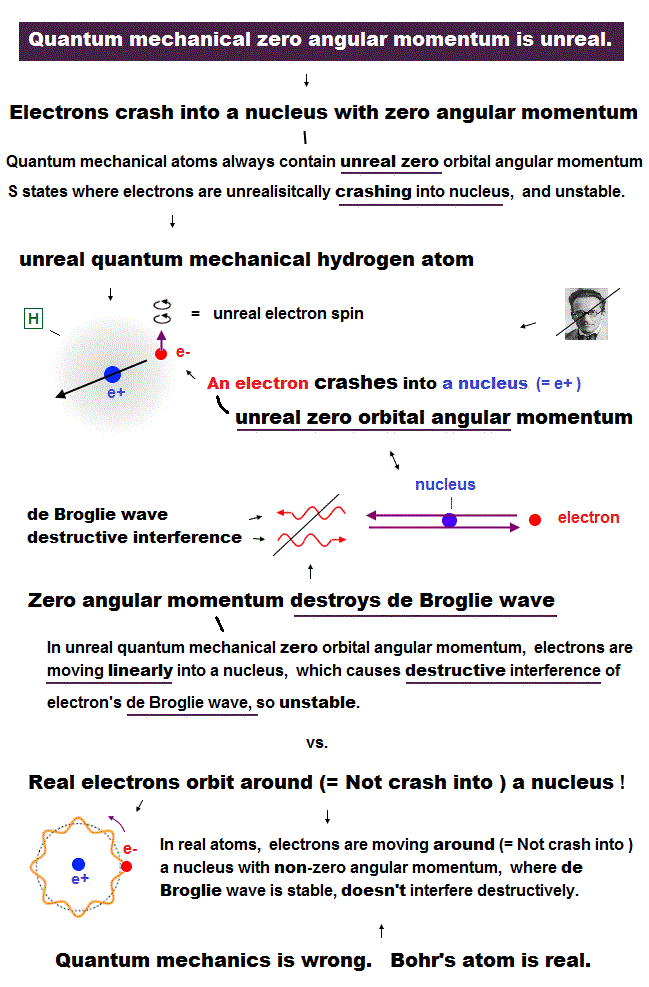Quantum mechanics claims an electron in hydrogen atom has zero orbital angular momentum.  It means the electron always crashes into nucleus in hydrogen ?

Furthermore, they claim Helium has two hydrogen-like orbitals (= 1s ) with zero angular momentum ( this p.3 below ).

It means two electrons in Helium also hit the nucleus ?  These quantum mechanical Helium models are clearly unstable and unrealistic, so wrong.

It's impossible that Schrodinger equation gets the "right" Helium wavefunction.  Instead, they just "choose" fake solution.

## Electron spin is a tiny magnet ?

### [ Spin is inconsistent with Pauli exclusion principle ! ]

(Fig.4)  Electron spin up and down cause magnetic field ?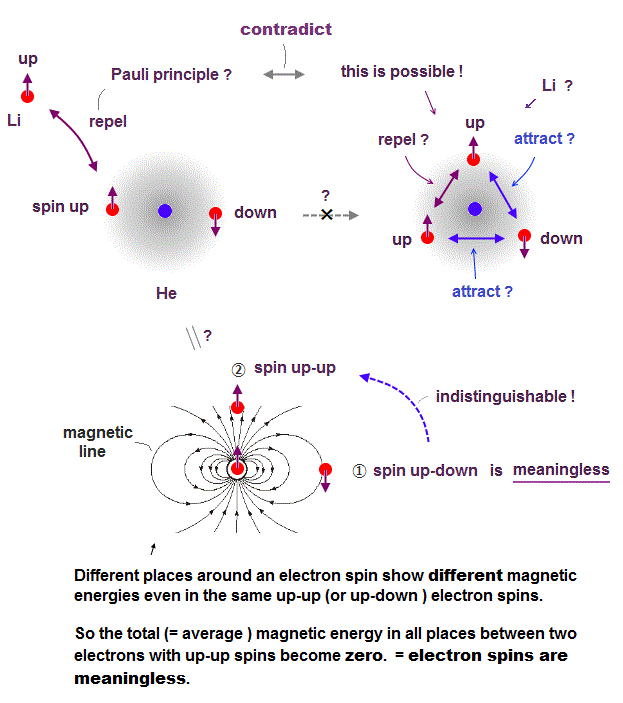In Stern-Gerlach experiment, they got some atoms through magnetic field to measure the magnitude of magnetic moment of each electron.

Surprisingly, the experiments showed that the magnitude of magnetic moment of electron spin is just equal to Bohr magneton in Bohr's classical orbit ( this p.4 )

We can safely say Stern-Gerlach experiment proved the magnetic property in Bohr's orbit ( Not unreaslitic spin ! )

## Spin-spin magnetic interaction is too weak !

### [ Spin cannot explain strong Pauli principle in Helium ! ]

(Fig.5)  Spin magnetic energy= 0.001 eV vs Pauli repulstion = 30 eVStern-Gerlach experiment showed electron spin has the magnetism equal to Bohr magneton.

From this measured magnetic moment, we can get the magnetic interaction between two electron spins.

This spin-spin magnetic energy is very weak (= about 0.001 eV ), compared with repulsive energy required for Pauli exclusion principle (= 30 eV ).

It means spin magnetic moment has nothing to do with Pauli exclusion principle, and they created artificial concept such as "exchange force" ( this p.7, this p.6 ).

After finding the magnitude of electron spin disagreed with strong Pauli principle, they fabricated artificial new force (= exchange ? ).  ← this is NOT a science !

## Helium with spins up and down  is false

### [ Two electrons cannot keep "up and down" spins ! ]

(Fig.6)  Depending on electrons' position, up-up spins are more stable !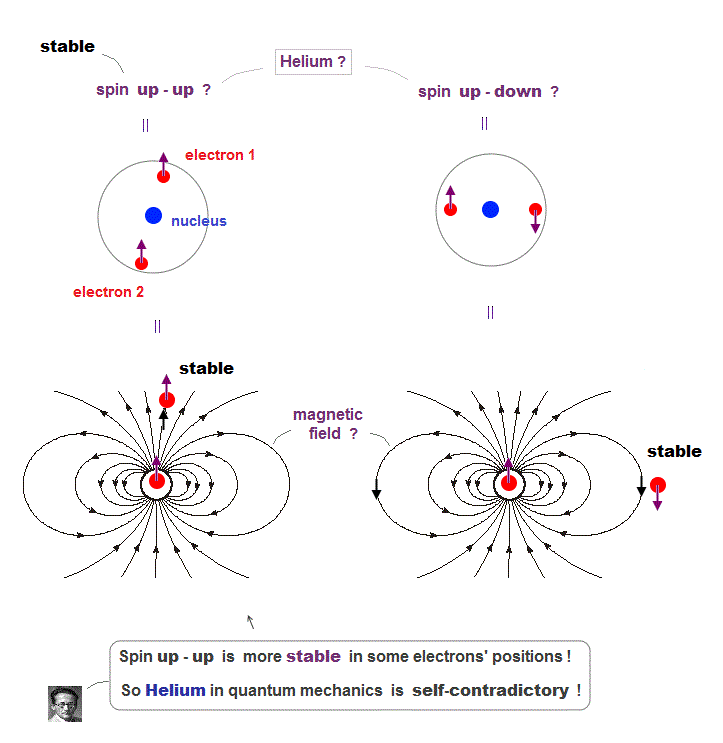Quantum mechanics claims Helium atom has a pair of "up" and "down" electron spins.  But in fact, this Helium model is inconsistent with electron's spin

They claim electron spin is a tiny magnet causing magnetic field aroud it.  Spin's directions change depending on two electrons' positional relasionship !

Magnetic source always includes a pair of N and S poles, which generates magnetic field lines around it.

To retuen from N to S pole, the magnetic field must include two opposite directions ( upward and downward ).

In Fig.6 left, when two spin directions are the same (= up-up ), they are more stable, because the spins are aligned along magnetic field !

So it's impossible to keep a pair of spins up-down of two Helium electrons.

## Electron de Broglie wave was confirmed.

### [ de Broglie relation was confimed in various experiments. ]

(Fig.7)  Double-slit experiments proved electron's de Broglie wavelength.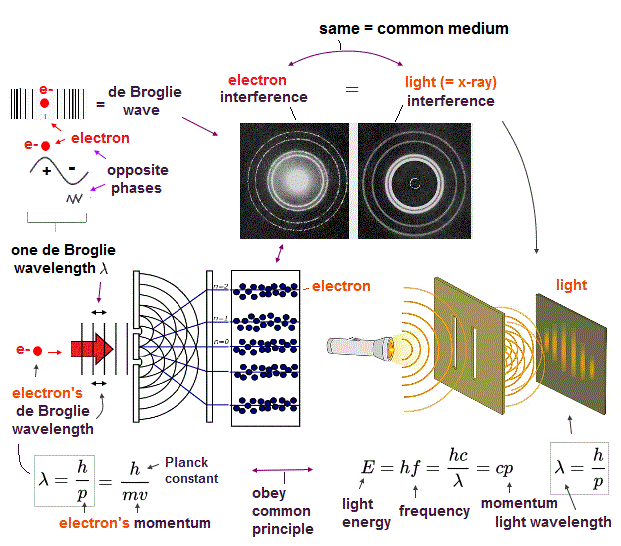In de Broglie relation, electron's wavelength λ is given by λ = h/mv, where m and v are electron's mass and velocity.

This important matter-wave relation was confirmed in various experiments such as doube-slit, Davisson-Germer and this.

So there is No room for doubt that this de Broglie wave is true.

In these experiments, an electron is driven out from destructive interference regions by the "force" of de Broglie wave ( this p.25 )

This strong "expelling force" by de Broglie wave interference can explain strong Pauli exclusion force !

## Opposite phases of de Broglie wave cancel each ohter

### [ Double-slit experiment showed "destructive interference" of electron's wave ]

(Fig.8)  Opposite de Broglie wave phases (= ± ) kick out an electron.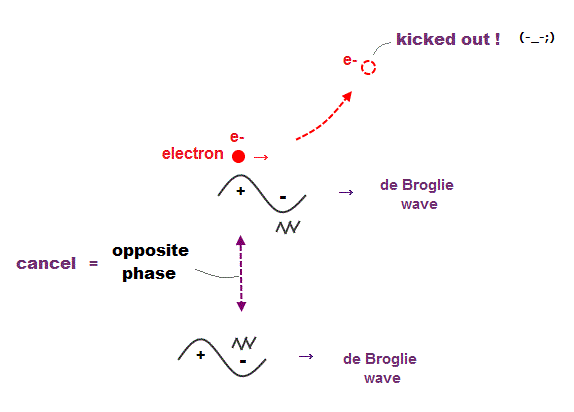Double-slit experiment showed an electron is de Broglie wave where the opposite wave phses (= ± ) cancel each other.

It means when the opposit wave phases interfere with each other "destructively", the electron is "kicked out" from it.

This strong evidence means the electron's de Broglie wave interference must be actually working in "real" Helium atom casing strong Pauli exclusion principle.

## An integer times de Broglie wavelength is needed

### [ to avoid destructive interference of de Broglie wave. ]

(Fig.9)  Quantum orbit satisfies an integer times de Broglie wavelength.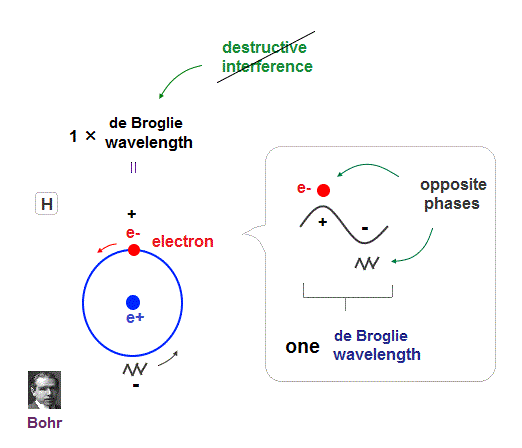Only Coulomb force is insufficient to explain why atomic energy levels are quantized, and why an electron doesn't fall into nucleus.

Bohr model succeeded in getting actual atomic energies, proposing each orbit is an integer times de Broglie wavelength, as well as Schrodinger's hydrogen .

An integer multiple of de Broglie wavelength means an electron can avoid destructive interference and be stable.

Without this de Broglie wave, each electron can be attracted to positive nuclei, until they stick to each other and its energy is unlimitedly lower !

So the repulsive force by electron's de Broglie wave is strong enough to keep the electron away from the nucleus and cause Pauli exclusion force.

## Schrodinger's Hydrogen, Helium are wrong !

### [ Angular momentum zero → de Broglie wave is destroyed ! ]

(Fig.10)  Electron's de Broglie wave in Schrodinger' hydrogen is destroyed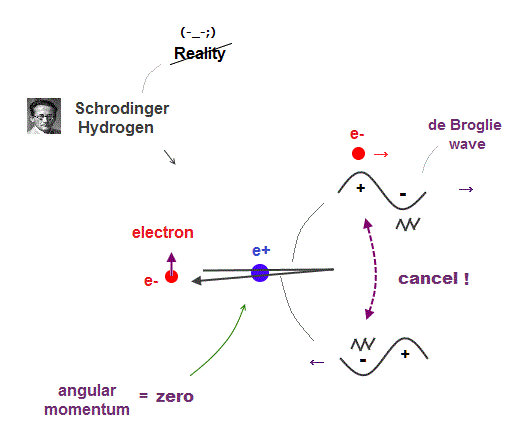Electron de Broglie wave with experimental proof contradicts quantum mechanical atomic model.

Quantum mechanics claims that electrons in Hydrogen and Helium have zero orbital angular momentum, which means "linear-shaped" orbital.

If so, the opposie phases in electron's de Broglie wave always overlap and interfere with each other "destructively" (= cancel ).

As you see, zero angular momentum in quantum mechanical atoms is inconsistent with de Broglie theory, so false.

## Why Old Bohr model Helium is wrong ?

### [ Old Bohr's Helium causes "destructive" interference. ]

(Fig.11)  Opposite phases of two de Broglie waves cancel each other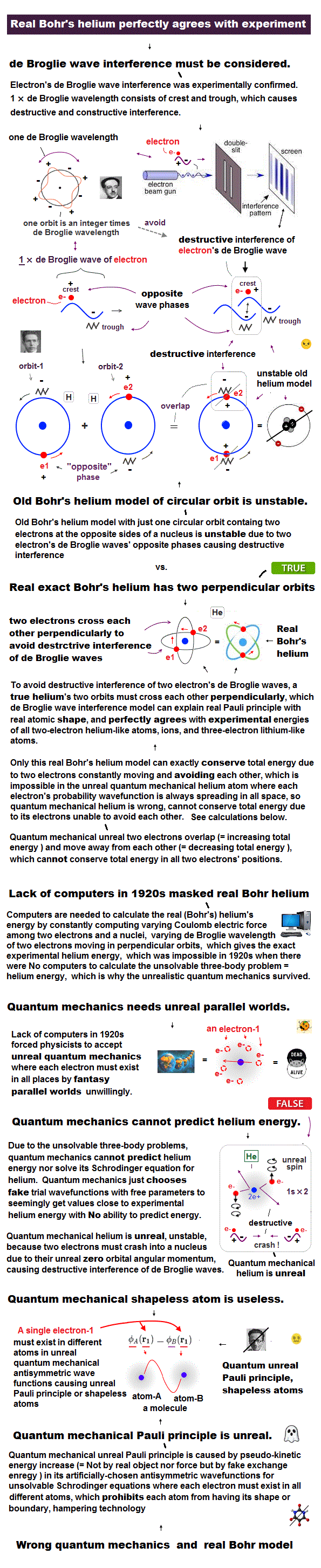In old Bohr's helium, two electrons are moving on the opposite sides of the nucleus in the same circular orbit (= one de Broglie wavelength ).

Considering Davisson-Germer interference experiment, two electrons of old Bohr's helium are clearly unstable.

1-de Broglie wavelength orbit consists of a pair of the opposite wave phases (= ±ψ ), which cancel each other by destructive interference.

Due to Coulomb repulsion between two electrons, one is always on the opposite side of another where the opposite de Broglie wave phases cancel each other.

Actually, old Bohr's helium of Fig.11 gives wrong ground state energy of helium, when you calculate it.

Old helium gives the total energy of -83.33 eV, which is a little lower than the actual value of -79.005 eV (= 1st + 2nd ionization energy of this ).

## de Broglie wave interference explains helium.

### [ Two de Broglie waves cross perpendicularly = stable. ]

(Fig.12)  Actual Helium must avoid destructive interference.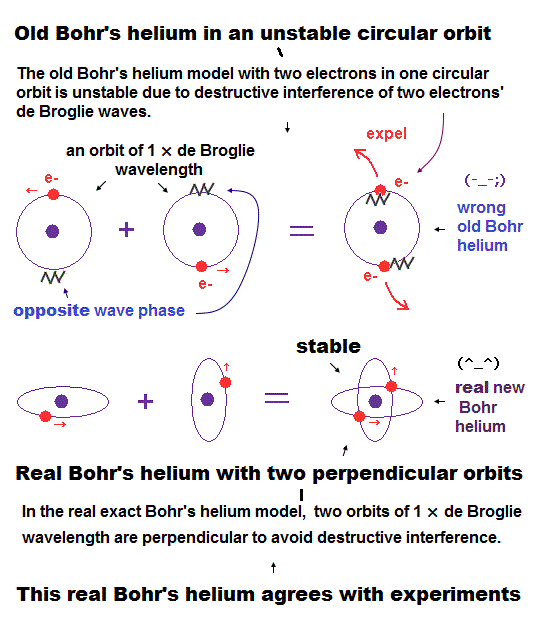If two 1 × de Broglie wavelength orbits are in the same plane in old Bohr's helium model, their opposite wave phases cause destructive interference and vanish.

To avoid vanishing de Broglie's wave, two electron orbits in actual helium must be perpendicular to each other.  Each orbit is one-de Broglie wavelength.

If the two orbits are perpendicular to each other, their wave phases are independent from each other and can be stable, not canceling each other.

This helium model considering actual de Broglie wave interference just agrees with experimental results of all atoms !

## New Bohr's helium satisfies 1 × de Broglie wavelength.

(Fig.13) Hydrogen and Helium atoms.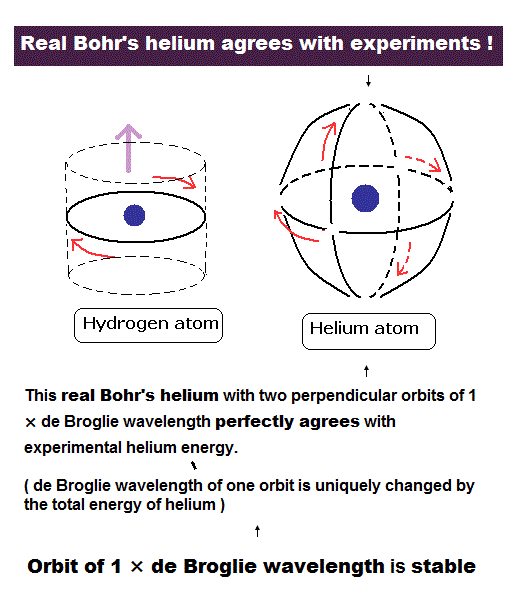These orbits are all just one de Broglie's wavelength.

In this new helium, the two symmetrical orbits crossing perpendicularly are wrapping the whole helium atom completely.

The Bohr model hydrogen which has only one orbit, can not wrap the direction of the magnetic moment completely.

It is just consistent with the fact of the strong stability and the closed shell property of helium.

## Why Helium has NO magnetic field ?

### [ Two opposite de Broglie waves cancel each other in Helium ]

(Fig.14)  Two "opposite" wave phases cancel each magnetic field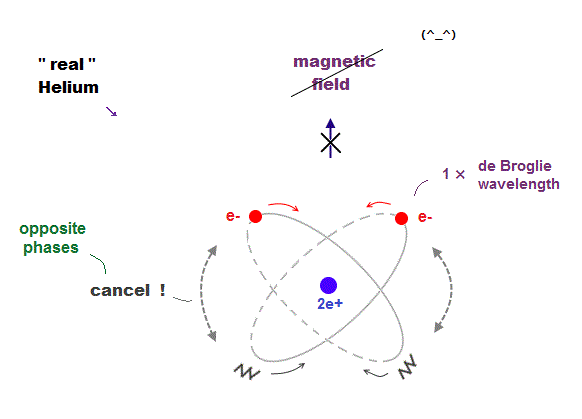One-electron Hydrogen generates magnetic field, while two-electron Helium doesn't.  Why ?  We can explain it using de Broglie waves.

In "actual" Helium model, the opposite phases of two electron's de Broglie waves cross each other perpendicularly.

Because two electrons repel each other by Coulomb repulsion, an electron tends to approch the opposite phase ( where electron doesn't exist ) of another electron.

From a distance, the total magnetic field caused by electron's movement (= de Broglie wave ) is cancelled out to be zero by destructive interference.

So crossing between the opposite de Broglie wave phases is the reason why Helium has NO magnetic field.

## Neon -- 2 × de Broglie wavelength

(Fig.15)  2 × de Broglie wavelength orbits. Each is a pair of opposite phases.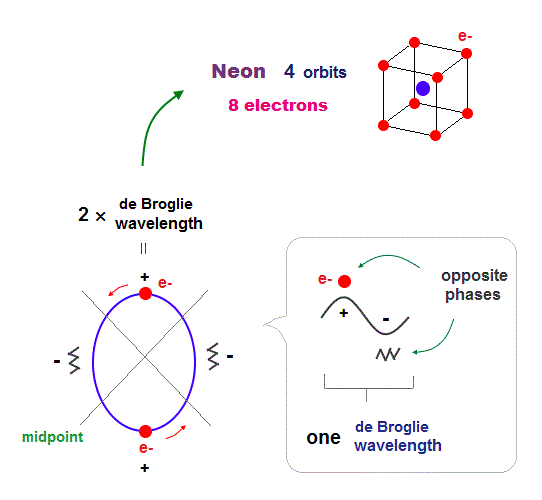One wavelength consists of a pair of "crest" (= + ) and "trough" (= - ) irrespective of transverse and longitudinal waves.

Here we suppose "+" phase contains an electron itself, and "-" phase is compressed by the electron's movement,   --- which is " de Broglie wave ".

2 × ( 1 × ) de Broglie wavelength orbit contains two ( one ) pairs of ±opposite phases and two ( one ) midpoint lines.

These "opposite" wave phases cancel each other by destructive interference.
To avoid it, two orbits must cross perpendicularly in all atoms.

## The periodic motions of eight valence electrons in Neon.

(Fig.16)  Orbits of Neon cross each other "perpendicularly".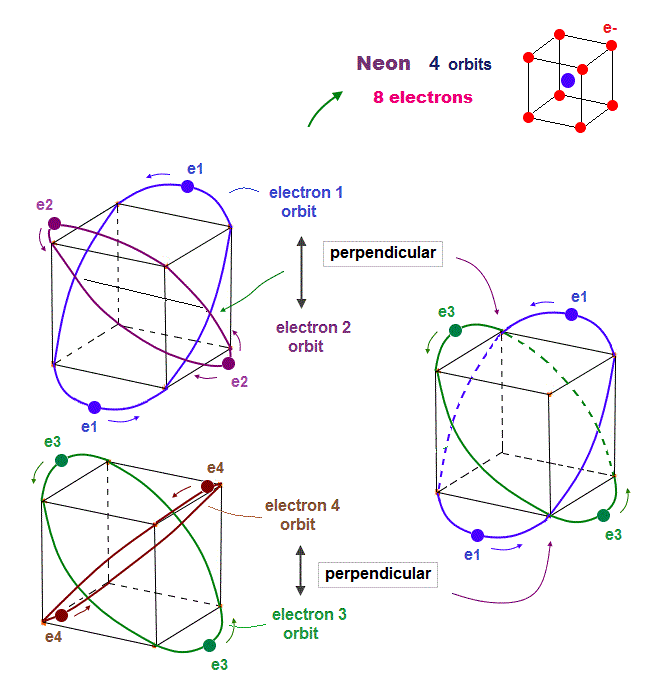(Fig.17)  Orbits of Neon cross each other "perpendicularly".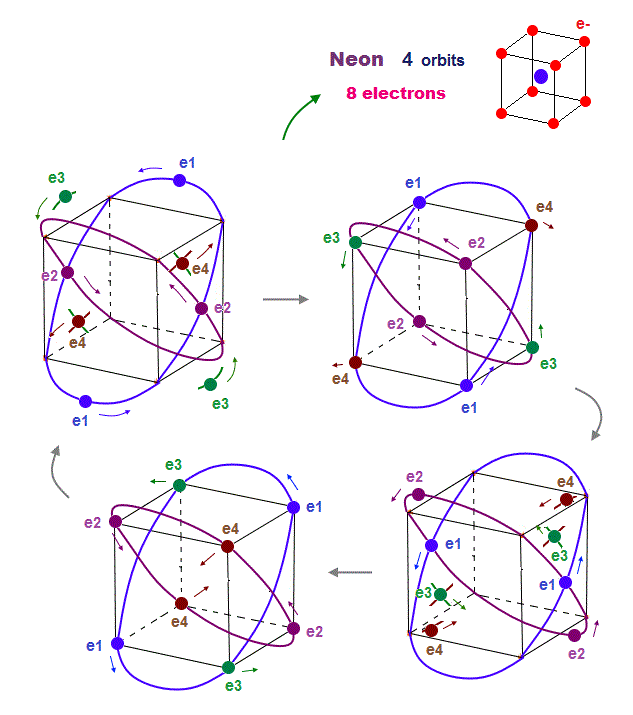As shown on this page, we can show the appropriate new Neon model, in which orbits can cross each other "perpendicularly".  "Perpendicular" crossing means they can avoid "destructive" interference.

## 8 electrons fill 2 × de Broglie wavelength orbits

### [ All 8 "opposite" wave phases cross each other in Neon. ]

(Fig.18)  Neon consists of 8 electrons (= 4 orbits with 2 wavelength )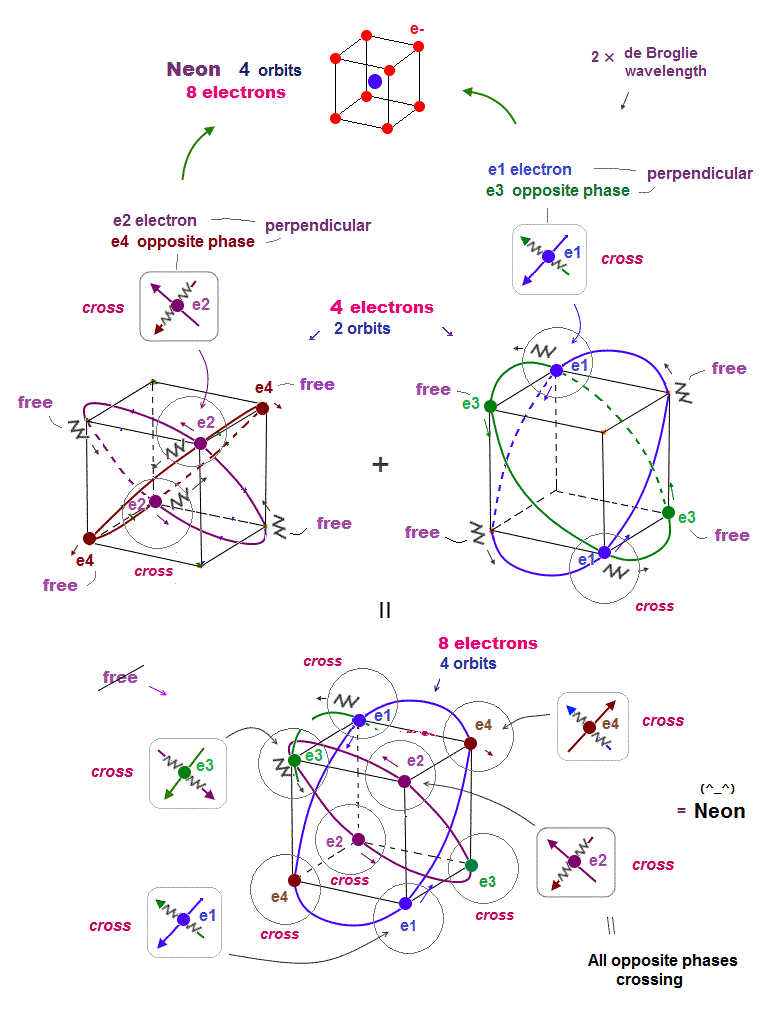Helium with 1 × de Broglie wavelength orbits can contain the maximum two electrons (= two orbits ).

In this case, all two pairs of the opposite wave phases (= ± ) cross each other.  In 2 × de Broglie wavelength orbit, Neon can contain 8 electrons (= 2 × 4 orbits ).

Because as shown in Fig.18 lower, if there are four orbits (= eight electrons ), all eight pairs of the opposite de Broglie wave phases just cross each other.

It means the maximum valence electron's number is "eight" in 2 × de Broglie wavelength Neon.  See this.2016/9/2 updated. Feel free to link to this site.